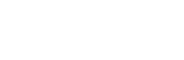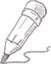1, 75%位数=上四分位数，50%位数=中位数 25%位数=下四分位数

2, 计算75%位数位置: 上四分位数的位置= (n+1) × 0.75=x.y 位置

= (10+1)*0.75

= 8.25位置

=24+(26-24)*0.25

=24.5

26.1312 7 0 关注作者 收藏

评论(0)